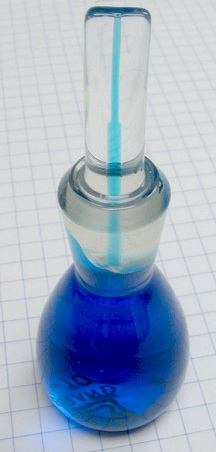# Difference Between Density and Specific Gravity

## Main Difference – Density vs. Specific Gravity

Density and specific gravity are both indications of how much mass a substance would occupy in a given volume. The main difference between density and specific gravity is that density is the mass per unit volume of the substance whereas specific gravity is a ratio comparing the density of one substance to the density of another reference substance.

## What is Density

Density of a substance refers to it mass per unit volume. Density is usually given the Greek letter “rho” ($\rho$). If the mass of a sample of the substance is$m$ and the volume of the sample is$V$, then the density is:$\rho=\frac{m}{V}$.

The SI unit for density is kg m-3. Often, other units are used to measure density in various fields of study. For instance in chemistry, grams per cubic centimetre (g cm-3) is used often to express density.

Density can change with temperature and pressure. Generally, density increases when subjected to increased pressure (particles making up the substance get squeezed into a smaller volume) and decreases when the temperature is increased (the substance expands and so the number of particles per unit volume becomes lower). However, water between 0 and 4 oC is a notable exception, because it counter-intuitively expands as the temperature is lowered within this range.

## What is Specific Gravity

Specific gravity is a ratio, comparing the density of a particular substance with the density of another reference substance, typically water. Specific gravity has no units. The apparent specific gravity of a substance is the ratio of the weight of a specific volume of a substance compared to the weight of the same volume of a reference substance. Apparent specific gravity is easily measured by measuring weights or by using a hydrometer. The true specific gravity, which refers to the ratio of densities of the two substances, need to be calculated from the measurements of apparent specific gravity.

pycnometer is a device that is used to measure the apparent specific gravity. It is usually a small glass container, which can be filled with the substance whose specific gravity is to be measured. The weight of the pycnometer also needs to be measured when it is empty, and again when it is filled with a reference substance. By using these measurements of weight, it is possible to calculate the apparent specific gravity and thereby the true specific gravity of the substance.A pycnometer

When using a hydrometer  to measure the specific gravity of a liquid, the hydrometer is dipped into he liquid. How far the hydrometer sinks down depends on the apparent specific gravity of the liquid, so the value of apparent specific gravity can be read off from a scale on the hydrometer.

## Difference Between Density and Specific Gravity

### What it Measures

Density measures the mass per unit volume of a substance.

Specific Gravity is a comparison of the density of a substance to the density of a reference substance.

### Dimensions

Density has dimensions [mass] [volume]-3. The SI unit for density is kg m-3.

Specific Gravity is dimensionless.

Image Courtesy

“A full pycnometer, photographed on 5 mm squares.” by Slashme (Own work) [Public Domain], via Wikimedia Commons

“Hydrometer for must: 0 … +130 °Oe (degrees Oechsle) at +20 °C” by Sönke Kraft aka Arnulf zu Linden (Own work) [CC BY-SA 3.0], via Wikimedia Commons